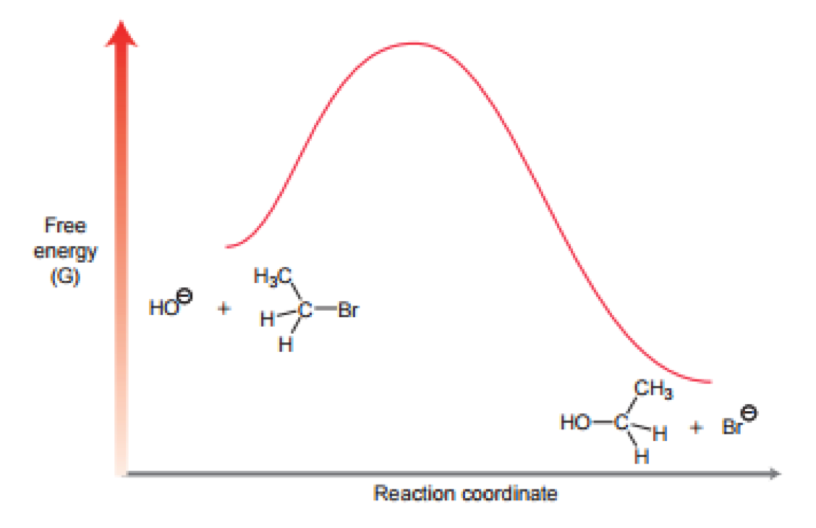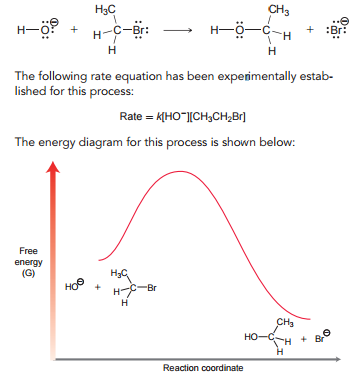Problem: Consider the following reaction:(h) Is the transition state closer in structure to the reactants or products? Explain.

FREE Expert Solution
84% (457 ratings)
FREE Expert Solution

We’re being asked to determine if the transition state for the reaction is closer in structure to the reactants or products. We’re given the following energy diagram:Recall that a transition state is the highest energy point in an energy diagram. According to the Hammond Postulate, transition states most closely resemble the species with the higher energy.

84% (457 ratings)
Problem Details

Consider the following reaction:

(h) Is the transition state closer in structure to the reactants or products? Explain.What scientific concept do you need to know in order to solve this problem?

Our tutors have indicated that to solve this problem you will need to apply the Hammond Postulate concept. You can view video lessons to learn Hammond Postulate Or if you need more Hammond Postulate practice, you can also practice Hammond Postulate practice problems .

What is the difficulty of this problem?

Our tutors rated the difficulty of Consider the following reaction:(h) Is the transition state ... as medium difficulty.

What professor is this problem relevant for?

Based on our data, we think this problem is relevant for Professor Boulos' class at BARRY.

What textbook is this problem found in?

Our data indicates that this problem or a close variation was asked in . You can also practice practice problems .